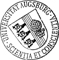## An Adaptive Newton Continuation Strategy for the Fully Implicit Finite Element Immersed Boundary Method

• The Immersed Boundary Method (IB) is known as a powerful technique for the numerical solution of fluid-structure interaction problems as, for instance, the motion and deformation of viscoelastic bodies immersed in an external flow. It is based on the treatment of the flow equations within an Eulerian framework and of the equations of motion of the immersed bodies with respect to a Lagrangian coordinate system including interaction equations providing the transfer between both frames. The classical IB uses finite differences, but the IBM can be set up within a finite element approach in the spatial variables as well (FE-IB). The discretization in time usually relies on the Backward Euler (BE) method for the semidiscretized flow equations and the Forward Euler (FE) method for the equations of motion of the immersed bodies. The BE/FE FE-IB is subject to a CFL-type condition, whereas the fully implicit BE/BE FE-IB is unconditionally stable. The latter one can be solved numerically byThe Immersed Boundary Method (IB) is known as a powerful technique for the numerical solution of fluid-structure interaction problems as, for instance, the motion and deformation of viscoelastic bodies immersed in an external flow. It is based on the treatment of the flow equations within an Eulerian framework and of the equations of motion of the immersed bodies with respect to a Lagrangian coordinate system including interaction equations providing the transfer between both frames. The classical IB uses finite differences, but the IBM can be set up within a finite element approach in the spatial variables as well (FE-IB). The discretization in time usually relies on the Backward Euler (BE) method for the semidiscretized flow equations and the Forward Euler (FE) method for the equations of motion of the immersed bodies. The BE/FE FE-IB is subject to a CFL-type condition, whereas the fully implicit BE/BE FE-IB is unconditionally stable. The latter one can be solved numerically by Newton-type methods whose convergence properties are dictated by an appropriate choice of the time step size, in particular, if one is faced with sudden changes in the total energy of the system. In this paper, taking advantage of the well developed affine covariant convergence theory for Newton-type methods, we study a predictor-corrector continuation strategy in time with an adaptive choice of the continuation steplength. The feasibility of the approach and its superiority to BE/FE FE-IB is illustrated by a representative numerical example.• Dokument_1.pdfAuthor: Ronald H. W. HoppeGND, Christopher LinsenmannGND urn:nbn:de:bvb:384-opus4-12043 https://opus.bibliothek.uni-augsburg.de/opus4/1495 Preprints des Instituts für Mathematik der Universität Augsburg (2011-03) Preprint English Universität Augsburg University of Houston 2011/03/08 finite element immersed boundary method; fully implicit scheme; predictor-corrector continuation; red blood cells Strömungsmechanik; Numerische Strömungssimulation; Finite-Elemente-Methode; Prädiktor-Korrektor-Verfahren Mathematisch-Naturwissenschaftlich-Technische Fakultät Mathematisch-Naturwissenschaftlich-Technische Fakultät / Institut für Mathematik Mathematisch-Naturwissenschaftlich-Technische Fakultät / Institut für Mathematik / Lehrstuhl für Numerische Mathematik 5 Naturwissenschaften und Mathematik / 51 Mathematik / 510 MathematikDeutsches Urheberrecht mit Print on Demand